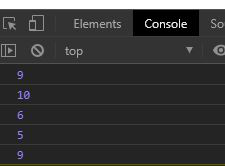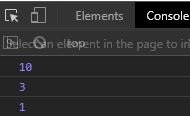Underscore.js _.random() Function

• Last Updated : 14 Jul, 2020

Underscore.js is a library in javascript that makes operations on arrays, string, objects much easier and handy.
The _.random() function is used to return a random integer which is in the range provided to the function.

Note: It is very necessary to link the underscore CDN before going and using underscore functions in the browser. When linking the underscore.js CDN The “_” is attached to the browser as a global variable.

Syntax:

_.random(min, max);

Parameters: It takes the following parameters.

• min value: It is the minimum random value to be returned by the function.
• max value: It is the maximum random value that can be returned by the function.

Return Value: It returns a random integer between the min and max. If only one value is passed then it will return random value between 0 and passed value.

Example 1:



Output:Example 2: If no parameter is given to the random function.



Output:Example 3: When only one parameter is given then random value is in range 0 to n, where n is the parameter given.



Output:My Personal Notes arrow_drop_up